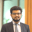Trusted answers to developer questions
Trusted Answers to Developer Questions

Related Tags

anime.js
javascript
animation

# How to round numerical values in Anime.jsRana Abdul Muneem

Grokking Modern System Design Interview for Engineers & Managers

Ace your System Design Interview and take your career to the next level. Learn to handle the design of applications like Netflix, Quora, Facebook, Uber, and many more in a 45-min interview. Learn the RESHADED framework for architecting web-scale applications by determining requirements, constraints, and assumptions before diving into a step-by-step design process.

### Overview

Anime.js is a JavaScript library used for creating animations. An element's properties like color, shape, size, and position can be animated, but sometimes we might need to animate numerical data such as the progress in a loading bar.

We can animate numerical data by accessing DOM attributes or (object properties if the target is a JavaScript object) as parameters of the anime() function.

Note: Click here if you don't know how to target objects in anime.js and here if you're unfamiliar with accessing attributes.

For a general overview of anime.js basics, click here.

Since JavaScript doesn't have an integer data type, it represents all numbers as floating point values. Anime.js provides the round property parameter to control the number of decimal places displayed.

### Syntax

The code below describes how to use the round parameter. The value is a power of 10 (1, 10, 100, ... ). This value controls how many decimal places to display.

anime({
...
round: value,
...
});
The syntax for using the round parameter in the anime() function

The value indicates the nearest position to round to. For example, if the value given is 1, the number is rounded to the nearest one's position. If the value given is 10, the number is rounded to the nearest tenth's position, and so on.

### Example

The example shows how to use the round parameter to animate numerical values to different decimal places.

Note: The following example uses the DOM property innerHTML to display the numbers. Click here to learn about the innerHTML property.

We consider three boxes, each with an increasing number. Each box has more decimal points from left to right than the last.

Using the round parameter for different decimal points

### Explanation

We create each box as a div element and create a function to animate each box in the JavaScript section of the code.

• Lines 1–7: We create an animation for the first box. We set the round value to 1. This value represents the 'ones' decimal place. In simple terms, the code will display no digits after the decimal point.
• Lines 8–14: We create the same animation for the second box. This time we set the round value to 10. This value represents the 10th decimal place. In other words, one digit will be displayed after the decimal point.
• Lines 15–21: We repeat the same animation for the third box. The round value is set to 100. So decimal places up to and including the 100th position will be displayed (2 decimal places).

RELATED TAGS

anime.js
javascript
animation

CONTRIBUTORRana Abdul Muneem